# You Need to Know Big O Notation: Here's Why

When we're programming, most of us think about solving the problem that lies ahead. But instead of asking, "How do I create code that works?" we should be asking, "How do I solve problems in the most efficient and elegant way, consuming as little processing capacity as possible?"

Why do we need to ask ourselves this question?

Because -- in addition to the software architecture, which helps to better structure code within the project, and the famous clean code that we should always use to maintain standard and easy-to-read code -- we have to be concerned about the efficiency of our code.

##### What is Big O?

How do we achieve efficiency? Meet Big O, which is pretty much a classification of the processing time our code can take. And as we know, processing is money, so the more efficient our code is, the cheaper the solution will be!

To help us understand what this Big O is, we're going to review a few math concepts, logarithm included (you didn't think you would ever use that again, right?). But there's no need to panic! We will take baby steps through these concepts and illustrate each with code as we go.First of all, we need to understand what time complexity is! Time complexity for software calculates how much time your code will take to run in relation to the size of the input of an algorithm, or its efficiency. So Big O is the calculation of time complexity.

##### O(1)

The first Big O Notation that we have is O(1). This simply means that the operation will always execute in a constant amount of time regardless of how big the input size is. It's a good method, because time does not grow in proportion to the size of the information entered.

Let's look at an example to illustrate this concept: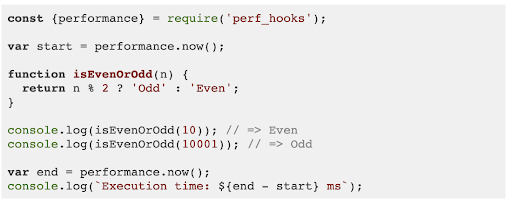The output for this is as follows:##### O(n)

Unlike O(1), O(n) time complexity grows in linear increments as the number of inputs increase. So Big O depends on the number of inputs.

For example: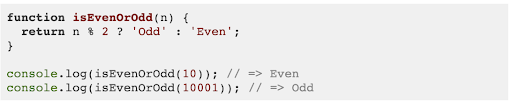And in this case, our output is as follows:##### O(n^2)

Like O(n), which grows proportionally, this one grows in proportion to the square of the input, usually when developers use nested loops inside their code.

Let's take a look: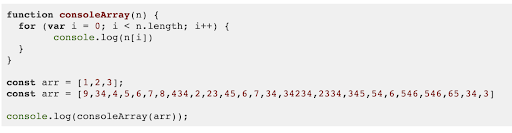With just 3 inputs on O(n^2), our output is as follows:But as we add inputs, you can see that the time of execution is going to increase more and more:##### O(2^n)

When the Big O reaches O(2^n), the time complexity growth doubles with each addition to the input data set. (This one is nooot good!)

Check out the example code to see what happens: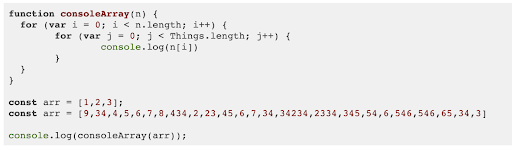So with 3 inputs on O(2^n), we have:But with just one more input, the execution time will double!##### O (log n)

Logarithmic time complexity is a lot more desirable than any other, because log(n) will barely experience any increase when the input doubles or triples.

To illustrate the point, let's look at an algorithm with time complexity O(log n), with 2 as the base number. You can see that the algorithm only runs 30 times when the input size is a trillion!

input size = 2 -> runs 1 time

input size = 4 -> runs 2 times

input size = 8 -> runs 3 times

input size = 16 -> runs 4 times

input size = 1,000,000,000,000 -> runs ~30 times

There are several ways to solve this problem, but the Logarithmic is the best way. For those who are familiar with the Fibonacci sequence, in the recursive version you couldn't get the 50th Fibonacci number.Let's take a look: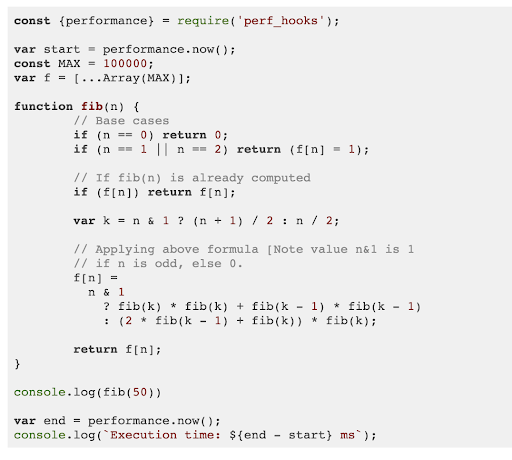Fibonacci 5th position execution example:Fibonacci 50th position execution example, where the execution time increased by only 1 second:This Big-O Complexity Chart illustrates each of the examples we've covered so far:Image courtesy of Jessica Yung

##### Conclusion

In this post, we learned how Big O Notation works. O(1) and O(log n) are the best options to use. So when the execution time of your code increases too much, now you'll be able to test its efficiency and understand why it is taking so long to process. To recap, when O(n^2) or O(2^n) are used and run with a big input, the processing time will be exponential.

There are other Big O notations that I did not cover in this article, but this is a helpful introduction to the concept!

I hope this article illustrated how important it is for you as a developer to understand the value of efficient code. And if you like this content, I encourage you to seek more knowledge about how your code performs and understand how to make it better.

##### References

Yang Bai. Big O Notation for Beginners: Understanding Code Efficiency. Medium.

Vibhor Thakral. A Beginner's Guide to the Big O Notation. hackernoon.

Alison Quaglia. A Beginner's Guide to Big O Notation (Part 1). Better Programming.

Changmin Lim. Big-O Notation for Beginners. Medium.

Program for Fibonacci numbers. GeeksforGeeks.

# Felipe Rezende

Felipe Rezende is a Software Developer at Avenue Code. He has worked with web applications for more than seven years, and he loves technology and is always trying to learn something new. Felipe is a supportive and enthusiastic team player who is dedicated to reducing processes and efficiently resolving project issues and programming problems.